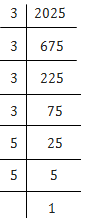# 2025 plants are to be planted in a garden in such a way that each row contains as many plants as the number of rows. Find the number of rows and the number of plants in each row.

Solution

Let as assume number of rows be, R

So, Each row has number of plants = R

Therefore, Total number of plants = R x R

That indcates R x R = 2025R2 = 3 × 3 × 3 × 3 × 5 × 5

R2 = (3 × 3) × (3 × 3) × (5 × 5)

R2 = (3 × 3 × 5) × (3 × 3 × 5)

R2 = 45 × 45

R = √(45 × 45)

R= 45

Therefore, The number of rows = 45

Also, the number of plants in each row = 45.